Graphical Method: FOPDT to Step Test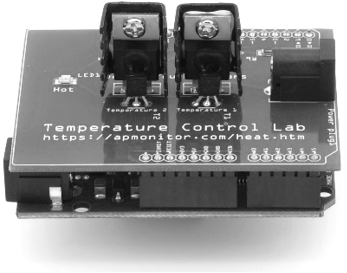Dynamic processes are often characterized by a gain (K_c), time constant (\tau_p), and sometimes dead-time (\theta_p). Use a graphical fitting method to estimate the three characteristic parameters of the following dynamic systems described by a first-order linear system with time delay.

$$\tau_p \frac{dy(t)}{dt} = -y(t) + K_p u\left(t-\theta_p\right)$$

For unstable or oscillatory responses, the first order linear equation does not represent the input to output relationship in the data. Indicate any system responses that are not a good fit for this equation form. Follow the following steps when fitting the parameters K_p, \tau_p, \theta_p to a step response.

1. Find \Delta y from step response
2. Find \Delta u from step response
3. Calculate K_p = {\Delta y} / {\Delta u}
4. Find \theta_p, apparent dead time, from step response
5. Find 0.632 \Delta y from step response
6. Find t_{0.632} for y(t_{0.632}) = 0.632 \Delta y from step response
7. Calculate \tau_p = t_{0.632} - \theta_p. This assumes that the step starts at t=0. If the step happens later, subtract the step time as well.

Problem 1Problem 2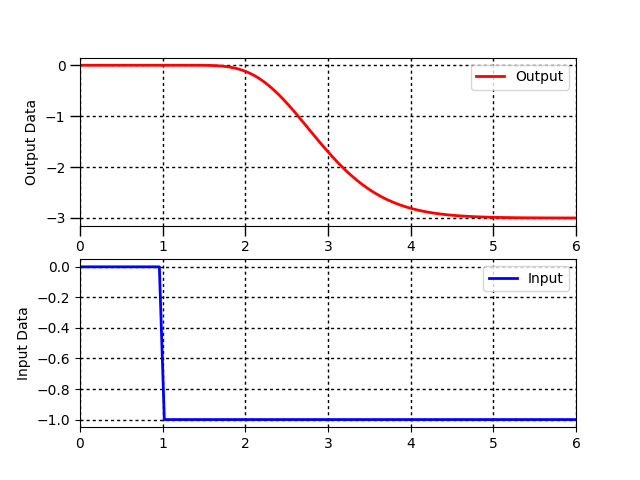Problem 3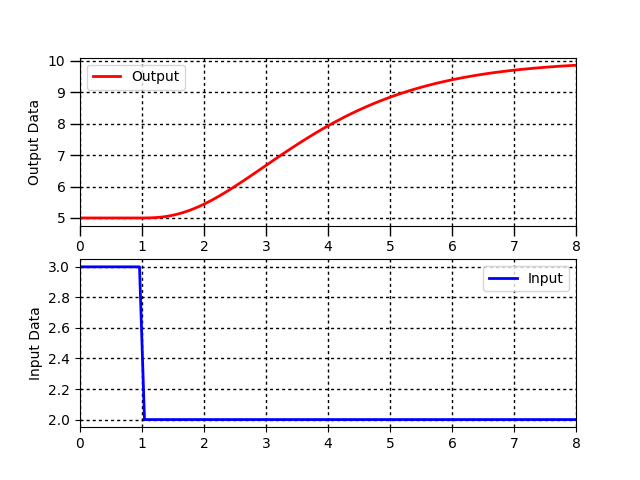Problem 4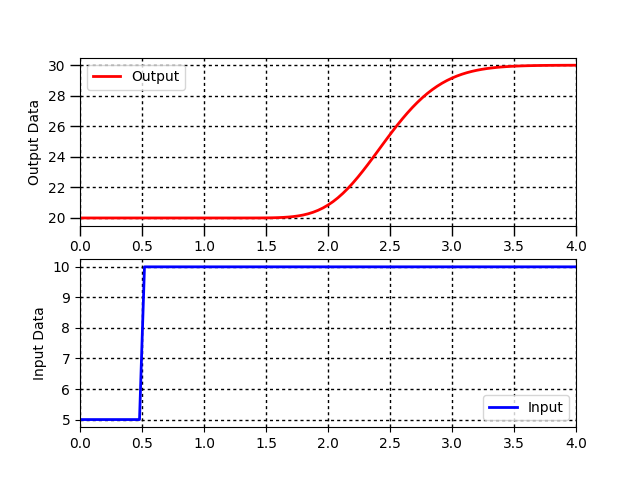Problem 5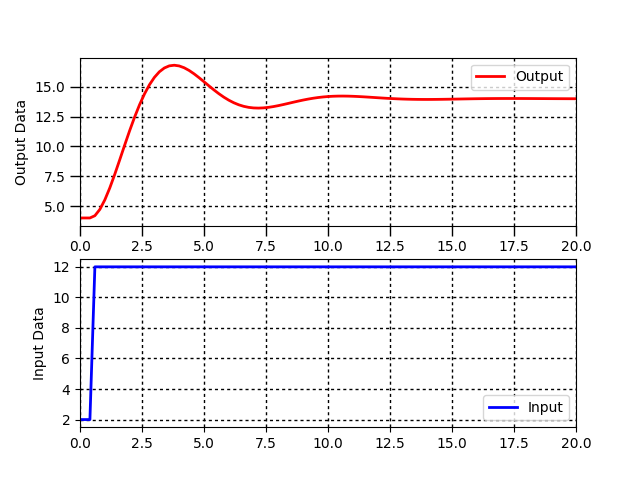Problem 6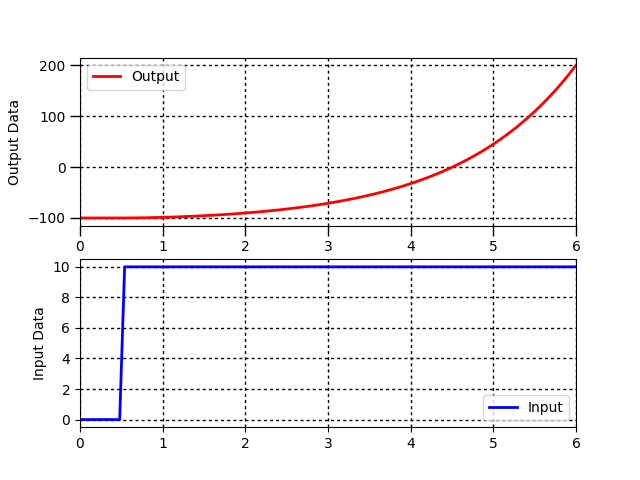Problem 7Solution